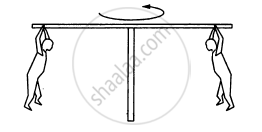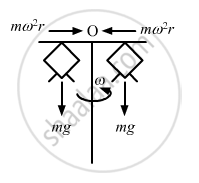Advertisement Remove all ads

# In a Children'S Park a Heavy Rod is Pivoted at the Centre and is Made to Rotate About the Pivot So that the Rod Always Remains Horizontal. Two Kids Hold the Rod Near the Ends - Physics

Sum

In a children's park a heavy rod is pivoted at the centre and is made to rotate about the pivot so that the rod always remains horizontal. Two kids hold the rod near the ends and thus rotate with the rod (In the following figure). Let the mass of each kid be 15 kg, the distance between the points of the rod where the two kids hold it be 3.0 m and suppose that the rod rotates at the rate of 20 revolutions per minute. Find the force of friction exerted by the rod on one of the kids.Advertisement Remove all ads

#### Solution

Given :

$\text {Frequency of rod }= n = 20 \text{ rev} \text{ per } \min$

$\Rightarrow \text{ n} = \frac{20}{60} = \frac{1}{3}\text{rev/s}$

Therefore, we have :
angular velocity of rod ,

$\text { Angular velocity of rod }= \omega = 2\pi n = \frac{2\pi}{3}\text{rad/s}$

Mass of each kid = $\text{m = 15 kg}$

Radius = $r = \frac{3}{2} = 1 . 5 \text{m}$$\therefore \text { Frictional force }= F = \text{mr }\omega^2$

$\Rightarrow F = 15 \times (1 . 5) \times \frac{(2\pi )^2}{9}$

$= 5 \times (0 . 5) \times 4 \pi^2 = 10 \pi^2 N$

Thus, the force of frictional on one of the kids is 10 $\pi$

Is there an error in this question or solution?
Advertisement Remove all ads

#### APPEARS IN

HC Verma Class 11, 12 Concepts of Physics 1
Chapter 7 Circular Motion
Q 23 | Page 115
Advertisement Remove all ads
Advertisement Remove all ads
Share
Notifications

View all notifications

Forgot password?